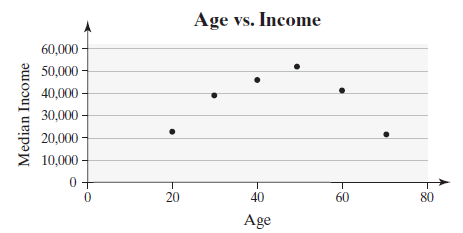×
Get Full Access to Statistics: Informed Decisions Using Data - 5 Edition - Chapter 4.1 - Problem 44
Get Full Access to Statistics: Informed Decisions Using Data - 5 Edition - Chapter 4.1 - Problem 44

×

# ?Faulty Use of Correlation On the basis of the scatter diagram on the next page, explain what is wrong with the following statement: “Because the lineaISBN: 9780134133539 240

## Solution for problem 44 Chapter 4.1

Statistics: Informed Decisions Using Data | 5th Edition

• Textbook Solutions
• 2901 Step-by-step solutions solved by professors and subject experts
• Get 24/7 help from StudySoup virtual teaching assistantsStatistics: Informed Decisions Using Data | 5th Edition

4 5 1 340 Reviews
13
3
Problem 44

Faulty Use of Correlation On the basis of the scatter diagram on the next page, explain what is wrong with the following statement: “Because the linear correlation coefficient between age and median income is 0.012, there is no relation between age and median income.”Step-by-Step Solution:

Step 1 of 5) Faulty Use of Correlation On the basis of the scatter diagram on the next page, explain what is wrong with the following statement: “Because the linear correlation coefficient between age and median income is 0.012, there is no relation between age and median income.” Making an Informed Decision. Suppose that you are in the market for a used car. To make an informed decision regarding your purchase, you decide to collect as much information as possible. What information is important in helping you make this decision See the Decisions project on page 178.

Step 2 of 2

## Discover and learn what students are asking

Calculus: Early Transcendental Functions : Graphs and Models
?In Exercises 5-14, sketch the graph of the equation by point plotting. y = 5 - 2x

Calculus: Early Transcendental Functions : Inverse Trigonometric Functions: Integration
?In Exercises 1-20, find the indefinite integral. $$\int \frac{t}{t^{4}+25} d t$$

Statistics: Informed Decisions Using Data : Properties of the Normal Distribution
?In Problems 25–28, the graph of a normal curve is given. Use the graph to identify the values of ? and ?.

Unlock Textbook Solution